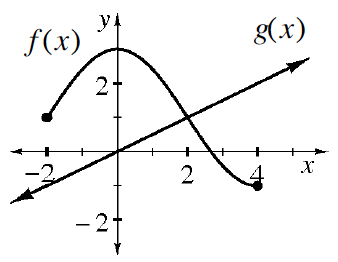### Home > INT1 > Chapter 11 > Lesson 11.2.5 > Problem11-109

11-109.

Examine the graph of functions $f(x)$ and $g(x)$ at right. Use the graph to approximate the values below (if possible).1. $f\left(0\right)$

When x is 0 in the relation f(x), what is y?

f(0) = 3

1. $g(4)$

When x is 4 in the relation g(x), what is y?

g(4) = 2

1. $f(2)$

See the hint for part (a).

1. $g(0)$

See the hint for part (b).

1. $g(2)$

See the hint for part (b).

1. $f(5)$

Is there a y-value for f(x) when x is 5?

1. $x\text{ such that}f(x)=g(x)$

For what $x$ value are the two functions equal?